# Improvements on Learning Kernel Extended Dictionary for Face Recognition

Improvements on Learning Kernel Extended Dictionary for Face Recognition

Corresponding Author Email:
yforghani@mshdiau.ac.ir
Page:
387-394
|
DOI:
https://doi.org/10.18280/ria.340402
22 June 2020
|
Accepted:
8 August 2020
|
Published:
30 September 2020
| Citation

OPEN ACCESS

Abstract:

Kernel extended dictionary learning model (KED) is a new type of Sparse Representation for Classification (SRC), which represents the input face image as a linear combination of dictionary set and extended dictionary set to determine the input face image class label. Extended dictionary is created based on the differences between the occluded images and non-occluded training images. There are four defaults to make about KED: (1) Similar weights are assigned to the principle components of occlusion variations in KED model, while the principle components of the occlusion variations have different weights, which are proportional to the principle components Eigen-values. (2) Reconstruction of an occluded image is not possible by combining only non-occluded images and the principle components (or the directions) of occlusion variations, but it requires the mean of occlusion variations. (3) The importance and capability of main dictionary and extended dictionary in reconstructing the input face image is not the same, necessarily. (4) KED Runtime is high. To address these problems or challenges, a novel mathematical model is proposed in this paper. In the proposed model, different weights are assigned to the principle components of occlusion variations; different weights are assigned to the main dictionary and extended dictionary; an occluded image is reconstructed by non-occluded images and the principle components of occlusion variations, and also the mean of occlusion variations; and collaborative representation is used instead of sparse representation to enhance the runtime. Experimental results on CAS-PEAL subsets showed that the runtime and accuracy of the proposed model is about 1% better than that of KED.

Keywords:

classification, sparse representation, kernel extended dictionary learning, occlusion

1. Introduction

Automatic face recognition (AFR) is one of the most important research topics in the field of machine vision, image processing and pattern recognition due to its wide range of applications. AFR is a very complicated and difficult process due to considerable intra-class variation, such as viewing angle, expression, lighting conditions, change of age, and occlusions. Occlusion can occur due to sunglasses, facial hair, wearing hat, etc.

One of the classification models which achieved great success in AFR is sparse representation for classification model (SRC) . The main idea of this minimization model is that an input face image can be expressed as a sparse linear combination of training images, and the larger coefficients of this linear combination belong to images that are in the same class as the input face image. Therefore, input image face class label can be determined. Compared with SRC, which assumes that noise also has a sparse representation, sparse representation on the basis of Maximum Correntropy is much more insensitive to outliers [2, 3]. Kernel sparse representation based classification (KSRC) algorithm [4, 5] maps data into a high dimensional feature space first and then SRC is performed in this new feature space by utilizing kernel trick. If the number of training data is low, KSRC may suffer from lack of training samples when mapping data from input data into feature space. To address this problem, a number of new training samples, termed virtual dictionary, are generated on the basis of the original training set to be used in feature mapping . The performance of SRC highly depends on the data distribution. SRC could not obtain satisfactory results on uncontrolled or imbalanced data sets. Sparse supervised representation classifier (SSRC) was proposed to solve these issues .

In extended SRC (ESRC), first, the intra-class variation of each face is determined based on standard and non-standard training set, and then, the input face image is expressed as a sparse linear combination of standard training images and intra-class variation . The accuracy of ESRC is better than that of the basic SRC but the runtime of ESRC is more than that of SRC. In collaborative representation for classification model (CRC), L2-norm is used instead of L1-norm of training data coefficients in the minimization model . Although this model cannot obtain a sparse representation of the input face image, but the accuracy of this model is not less than the basic SRC and its runtime is better than that of basic SRC. Akhtar et al.  showed that fusion of SRC and CRC can increase the accuracy. In Locally Linear K-Nearest Neighbers (LLK) , higher priority is assigned to nearest neighbors of input face image for contributing in linear representation of that input face image. The accuracy of LLK is better than that of basic SRC. Kernel extended dictionary learning model (KED)  is a new type of ESRC. In this model, first, training data features are extracted using local binary patterns (LBP) . The preprocessed training data then are mapped into a High-dimensional feature space. Next, by using Kernel Discriminant Analysis (KDA) , standard training images are mapped into a space with the least intra-class variation and inter-class similarity. Then, the main directions of occlusion variations are determined according to the differences between the standard training set and occluded training set in the high-dimensional feature space using Kernel Principle Component Analysis (KPCA) , and are transformed into the KDA space. Finally, the input face image, whose features are extracted by LBP, are mapped into the KDA space, and represented as a sparse linear combination of standard training set in the KDA space (basic dictionary) and the main directions of occlusion variations determined by KPCA and transformed into the KDA space (Extended Dictionary).

There are four defaults to make about KED: (1) Similar weights are assigned to the principle components of occlusion variations in KED model, while the principle components of the occlusion variations have different weights, which are proportional to the principle components Eigen-values. (2) Reconstruction of an occluded image is not possible by combining only non-occluded images and the principle components (or the directions) of occlusion variations, but it requires the mean of occlusion variations. (3): The importance and capability of main dictionary and extended dictionary in reconstructing the input face image is not the same, necessarily. (4) KED Runtime is high. To address these problems or challenges, a novel mathematical model is proposed in this paper. In the proposed model, different weights are assigned to the principle components of occlusion variations; different weights are assigned to the main dictionary and extended dictionary; an occluded image is reconstructed by non-occluded images and the principle components of occlusion variations, and also the mean of occlusion variations; and collaborative representation is used instead of sparse representation to enhance the runtime. Experimental results on CAS-PEAL subsets showed that the runtime and accuracy of the proposed model is about 1% better than that of KED.

In continue, SRC, CRC, ESRC and KED is described in Section 2, and the proposed model is presented in Section 3. In Section 4, experimental results are reported, and then conclusion is drawn in Section 5.

2. Prerequisites

2.1 SRC

Consider the training set $X=\left[\mathrm{x}_{1}, \mathrm{x}_{2}, \ldots, \mathrm{x}_{\mathrm{n}}\right]$, in which $\mathrm{x}_{\mathrm{i}} \in \mathrm{R}^{\mathrm{d}}$. The class label of $x_i$ is $c_{i} \in[1, C]$. To determine the label of input face image $\mathrm{y} \in \mathrm{R}^{\mathrm{d}}$, based on the training data X, the input face image y  are first represented as a sparse linear combination of training set by the following mathematical model :

$\min _{\beta}\|\mathrm{y}-\mathrm{X} \beta\|^{2}+\gamma\|\beta\|_{1}$          (1)

where, γ≥0 is user-determined parameter of the model which determines the importance of the second term of the model (1) with respect to its first term. The first term of the model (1) represent the input face image y as a linear combination of training set, where βi is the coefficient of i-th training data in this linear combination. Since there are several representations for the input face image y, the second term of the model (1) selects the sparse linear combination among these linear combinations. In fact, $\gamma$ controls the sparseness of this linear combination. It is expected that for a proper $\gamma$, the input face image y is represented as linear combination of a small number of training data of the same class as y. Model (1) can be solved by different algorithms, such as FISTA . Let δl(β) be a vector whose i-th entry is equal to βi if ci is equal to l, otherwise its i-th element is equal to zero. ci is the class label of i-th face image. The class label of the input data y is l* which minimizes the amount of the following residual:

$r_{l^{*}}(y)=\left\|y-X \delta_{l^{*}}(\beta)\right\|^{2}$           (2)

2.2 CRC

The SRC model is not differentiable. Therefore, fast gradient based algorithm cannot be used to solve SRC. To address this problem, CRC model was proposed. CRC model is as follows:

$\min _{\beta} F=\|y-X \beta\|^{2}+\gamma\|\beta\|^{2}$      (3)

The objective function of CRC model, unlike the SRC model, is differentiable. Therefore, to solve the model (3), it is enough to solve the following equation:

$\frac{\partial F}{\partial \beta}=0$      (4)

We have

$0=\frac{\partial F}{\partial \beta}=-2 X^{T}(y-X \beta)+2 \gamma \beta \\ \rightarrow \beta=Z y$                (5)

where,

$Z=\left(X^{T} X+\gamma I\right)^{-1} X^{T}$       (6)

Eq. (6) is calculated only once in the training phase, and the coefficient vector β is calculated just by multiplication of the matrix Z and the input data y, while in the SRC model, to calculate β, the SCR model must be solved for each input face image y using FISTA algorithm. Therefore, the CRC method is faster than the SRC.

2.3 ESRC

Both SRC and CRC are not robust to non-standard face images. To solve this problem, ESRC was proposed. Suppose that training face images include two types of face images: standard face images and non-standard face images. Standard images are obtained under standard conditions, for example, standard lighting conditions, standard viewing angle, standard expression, and without glasses. Let $\widetilde{\mathrm{X}}=\left[\tilde{\mathrm{x}}_{1}, \tilde{\mathrm{x}}_{2}, \ldots, \tilde{\mathrm{x}}_{\widetilde{n}}\right]$ be the non-standard face images and $\mathrm{X}=\left[\mathrm{x}_{1}, \mathrm{x}_{2}, \ldots, \mathrm{x}_{\mathrm{n}}\right]$ be standard ones. Also, let $\tilde{\mathrm{X}}_{\mathrm{i}}$ be the non-standard face image of i-th individual and $\mathrm{X}_{\mathrm{idx}(\mathrm{i})}$ be his standard face image. Therefore, intra-class variation bases are as follows:

$\tilde{E}=\left[\tilde{x}_{1}-x_{i d x(1)}, \tilde{x}_{1}-x_{i d x(2)}, \ldots, \tilde{x}_{\tilde{n}}-x_{i d x(\tilde{n})}\right]$

where, $\tilde{\mathrm{n}}$ is the number of non-standard images. In ESRC, the input face image y is represented as sparse linear combination of standard images and intra-class variation using the following model:

$\min _{\beta, \tilde{\beta}}\left\|y-[X, \tilde{E}]\left[\begin{array}{c}\beta \\ \tilde{\beta}\end{array}\right]\right\|^{2}+\gamma\left\|\left[\begin{array}{c}\beta \\ \tilde{\beta}\end{array}\right]\right\|_{1}$             (7)

Finally, the label of the input face image y is equal to the class label $l^{*}$ which minimizes the following residual:

$r_{l^{*}}(y)=\left\|y-[X, \tilde{E}]\left[\begin{array}{c}\delta_{l^{*}}(\beta) \\ \tilde{\beta}\end{array}\right]\right\|^{2}$               (8)

2.4 Kernel extended dictionary (KED)

ESRC has better generalization ability than SRC, but its runtime time is highly influenced by the number of the intra-class variation bases $\widetilde{\mathrm{E}}$. One of the goals of KED is to address this problem.

2.4.1 Learning basic dictionary

In KED, all training data except the occluded face images are first transformed into a C-1-dimensional space with the least intra-class variation and inter-class similarity using KDA. Let $\widetilde{\mathrm{V}}=\left[\tilde{\mathrm{v}}_{1}, \tilde{\mathrm{v}}_{2}, \ldots, \tilde{\mathrm{v}}_{\mathrm{C}-1}\right]$ be C-1 axes in the (C-1)-dimensional KDA space, and

$\tilde{v}_{i}=\sum_{j=1}^{n} \tilde{a}_{i j} \varphi\left(x_{j}\right)$        (9)

where, φ is a mapping function which maps data from input space into a high-dimentional feature space, and $\tilde{a}_{i}=\left[\tilde{a}_{i 1}, \tilde{a}_{i 2}, \ldots, \tilde{a}_{i n}\right]$ is a vector obtained using KDA. Then, the input face image y and the standard training images in the KDA space (the basic dictionary) which are denoted by $\mathrm{y}_{\mathrm{KDA}}$ and $\widetilde{D}$, respectively, are calculated as follows:

$y_{K D A}=\tilde{V}^{T} \varphi(y)=\left[\tilde{a}_{1}^{T} K(y), \tilde{a}_{2}^{T} K(y), \ldots, \tilde{a}_{C-1}^{T} K(y)\right]^{T}$            (10)

$\tilde{v}_{i}=\sum_{j=1}^{n} \tilde{a}_{i j} \varphi\left(x_{j}\right)$       (11)

where,

$K(y)=\left[k\left(\tilde{x}_{1}, y\right), k\left(\tilde{x}_{2}, y\right), \ldots, k\left(\tilde{x}_{\tilde{n}}, y\right)\right]^{T}$          (12)

and $\mathrm{k}(., .)$ is Gaussian kernel function.

2.4.2 Learning the model of occluded face images or extended dictionary

The occluded face images were not used to learn basic dictionary model by KDA because an occlusion in a face images causes a broad change in the face image, and an occluded face image is considered to be an outlier in KDA. When there is outlier in training set, KDA cannot find a proper space with the least intra-class variation and inter-class similarity.

Let $\dddot{X}=\left[\dddot{x}_{1}, \dddot{x}_{2}, \dots, \dddot{x}_{\dddot{n}}\right]$ be occluded face images. Occlusion variation in high-dimensional feature space is as follows:

$\dddot{E}=\left[\begin{array}{c}\varphi\left(\dddot{x}_{1}\right)-\varphi\left(x_{i d x(1)}\right), \\ \left.\varphi\left(\dddot{x}_{2}\right)-\varphi\left(x_{i d x(2)}\right)\right) \\ \cdots \\ \varphi\left(\dddot{x}_{i n}\right)-\varphi\left(x_{i d x(\dddot{n})}\right)\end{array}\right]$        (13)

where, x(idx(i)) is the standard image of the occluded image of i-th individual $p$ principle components of the occlusion variation which are denoted by $\mathrm{V}^{\cdots}=\left[\mathrm{v}^{\cdots} _1, \mathrm{v} \cdots _2, \ldots, \mathrm{v} \cdots _\mathrm{p}\right]$, can be obtained using KPCA model, namely the following model:

$\max _{\dddot{V}} \dddot{V}^{T} S \dddot{V} \\ subject to \dddot{V}^{T} \dddot{V}=I$         (14)

where, I is identity matrix, and

$S=\sum_{i=1}^{\dddot{n}} \varphi_{i} \varphi_{i}^{T}$       (15)

where $\varphi_{\mathrm{i}}=\varphi\left(\dddot{\mathrm{x}}_{\mathrm{i}}\right)-\varphi\left(\mathrm{x}_{\mathrm{idx}(\mathrm{i})}\right)$. The constraints of the model (14) enforce the length of occlusion variation principle components to be equal to 1. To solve the model (14), the following eigen-vector problem must be solved:

$S \dddot{V}=\Lambda \dddot{V}$       (16)

where, Λ is a matrix of which main diagonal elements are the eigen-values corresponding to the eigen-vector matrix $\dddot{V} \cdot \dddot{V}$ contains the p more important eigenvectors of the matrix S.

We have:

$\dddot{v}_{i}=\sum_{j=1}^{\dddot{n}} \dddot{a}_{i j} \varphi_{j}$        (17)

where, $\dddot{a}_{i j} \in R$. Therefore, the problem (16) can be written as follows:

$\dddot{K} \dddot{a}=\dddot{\Lambda} \dddot{a}$       (18)

in which,

$\dddot{K}_{i j}=\varphi_{i}^{T} \varphi_{j} \\ =\left(\varphi\left(\dddot{x}_{i}\right)-\varphi\left(x_{i d x(i)}\right)\right)^{T}\left(\varphi\left(\dddot{x}_{i}\right)-\varphi\left(x_{i d x(i)}\right)\right) \\ =k\left(\dddot{x}_{i}, \dddot{x}_{i}\right)+k\left(x_{i d x(i)}, x_{i d x(i)}\right)-2 k\left(\dddot{x}_{i}, x_{i d x(i)}\right)$          (19)

and $\ddot{\Lambda}$ is the matrix of which main diagonal elements are the eigen-values corresponding to the eigenvector matrix $\dddot{a}$. After solving the problem (18) and obtaining optimal value of $\dddot{a}$, p principle components of the occlusion variation $\dddot{E}$ are determined using Eq. (17). Then, the principle components of the occlusion variation in KDA space (Extended Dictionary) which are denoted by $\dddot{D}$, are obtained by projecting the principle components of occlusion variation $\dddot{V}$ on the KDA space $\widetilde{V}$ as follows:

$\dddot{D}=\tilde{V}^{T} \dddot{V}$        (20)

where,

$\dddot{D}_{i l}=\tilde{v}_{i}^{T} \dddot{v}_{l} \\ =\left(\sum_{j=1}^{n} \tilde{a}_{i j} \varphi\left(x_{j}\right)\right)^{T}\left(\sum_{j=1}^{\dddot{n}} \dddot{a}_{l t} \varphi_{t}\right) \\ =\left(\sum_{j=1}^{n} \tilde{a}_{i j} \varphi\left(x_{j}\right)\right)^{T}\left(\sum_{j=1}^{\dddot{n}} \dddot{a}_{l t}\left(\varphi\left(\dddot{x}_{t}\right)-\varphi\left(x_{i d x(t)}\right)\right)\right) \\ =\tilde{a}_{i}^{T} \widetilde{K} \dddot{a}_{l}$            (21)

$\tilde{K}_{i j}=k\left(x_{i}, \dddot{x}_{j}\right)-k\left(x_{i}, x_{i d x(j)}\right)$         (22)

Finally, the input face image y in the KDA space denoted by $\mathrm{y}_{\mathrm{KDA}}$  is represented as a linear combination of the basic dictionary $\widetilde{D}$ and the extended dictionary $\dddot{D}$ using the following model:

$\min _{\widetilde{\beta}, \dddot{\beta}}\left\|y_{K D A}-[\widetilde{D}, \dddot{D}]\left[\begin{array}{c}\tilde{\beta} \\ \dddot{\beta}\end{array}\right]\right\|^{2}+\gamma\left\|\left[\begin{array}{c}\tilde{\beta} \\ \dddot{\beta}\end{array}\right]\right\|_{1}$         (23)

Eventually, the class label of the input face image y is equal to $1^{*}$ which minimizes the following residual:

$r_{l^{*}}(y)=\left\|y_{K D A}-[\widetilde{D}, \dddot{D}]\left[\begin{array}{c}\delta_{l}(\tilde{\beta}) \\ \dddot{\beta}\end{array}\right]\right\|^{2}$         (24)

3. Our Proposed Model

There are four criticisms to make about KED:

• First criticism: Similar weights are assigned to the principle components of occlusion variations in KED model, while the principle components of occlusion variations have different weights, which are proportional to the principle component eigen-values. If the eigen-value of a principle component is high, occlusion variation along its corresponding eigen-vector is considerable. Therefore, variation along this eigen-vector is more probable than an eigen-vector with a small eigen-value. Thus, unlike KED, we must assign a higher priority to variation along an eigen-vector with a large eigen-value with respect to variation along an eigen-vector with a small eigen-value in our model.
• Second criticism: An occluded face image is the sum of its corresponding non-occluded face image and occlusion. Reconstruction of an occluded image is not possible by combining only non-occluded images and the principle components (or the directions) of occlusion variations, but it requires the mean of occlusion variations because reconstruction of an occlusion is not possible by only the principle components of occlusion variations. For further explanation, we showed the occlusion, i.e. the difference between an individual's occluded and non-occluded face image, with a point in Figure 1. Directions or the principle components of occlusion variation were shown with two lines in Figure 1. These directions are determined using KPCA. According to the constraints of KPCA, i.e. the model (14), the length of principle components is the same. In KED, the p most important components of the principle components of occlusion variation are selected. Let p=1. Therefore, the most important direction is only selected from the two main directions specified in Fig. 1. This principle component or the most important direction was shown with a solid line in Figure 1. Obviously, it is not possible to reconstruct the occlusions or the points shown in Figure 1 with this principle component, i.e. the most important direction. In other words, a specified occlusion with a point in Figure 1 cannot be reached by moving from the origin along the most important direction. In order to reconstruct the occlusions or the points shown in Figure. 1, we must move from the occlusion mean along the most important direction.

## 1.png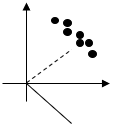Figure 1. Occlusion cannot be reconstructed using the most important principle component of occlusion variation. Each point represents an occlusion, and each line is a principle component of occlusion variation

• Third criticism: The importance and capability of main dictionary and extended dictionary in reconstructing the test image is not the same, necessarily, while their importance is considered to be the same in KED.
• Fourth criticism: The runtime of sparse representation-based classification used in KED model is more than collaborative representation.

To address these four problems or challenges, we propose the following model:

$\min _{\bar{\beta}, \dddot{\beta}} F=\left\|y_{K D A}-[\overline{\bar{D}}, \theta \dddot{D} \dddot{\Lambda}]\left[\begin{array}{c}\overline{\bar{\beta}} \\ \dddot{\beta}\end{array}\right]\right\|_{2}^{2}+\gamma\left\|\left[\begin{array}{c}\overline{\bar{\beta}} \\ \dddot{\beta}\end{array}\right]\right\|_{2}^{2}$             (25)

where, $\overline{\bar{D}}$ is the basic dictionary $\widetilde{D}$ together with the occluded images mean in the KDA space which are denoted by $\mu_{\mathrm{KDA}}$, i.e.

$\overline{\bar{D}}=\left[\widetilde{D}, \mu_{K D A}\right]$       (26)

$\mu_{\mathrm{KDA}}=\widetilde{\mathrm{V}}^{\mathrm{T}}\left(\frac{1}{\dddot{\mathrm{n}}} \sum_{\mathrm{j}=1}^{\dddot{\mathrm{n}}} \varphi_{\mathrm{j}}\right) \\ =\frac{1}{\dddot{\mathrm{n}}} \widetilde{\mathrm{V}}^{\mathrm{T}} \sum_{\mathrm{j}=1}^{\dddot{\mathrm{n}}} \varphi\left(\dddot{\mathrm{x}}_{\mathrm{j}}\right)-\varphi\left(\mathrm{x}_{\mathrm{idx}(\mathrm{j})}\right) \\=\frac{1}{\dddot{\mathrm{n}}}\left[\tilde{a}_{1}^{T} \sum_{j=1}^{\dddot{\mathrm{n}}}\left(K\left(\dddot{x}_{j}\right)-K\left(x_{i d x(j)}\right)\right), \ldots\right. \\ \left.\tilde{a}_{C-1}^{T} \sum_{j=1}^{\dddot{\mathrm{n}}}\left(K\left(\dddot{x}_{j}\right)-K\left(x_{i d x(j)}\right)\right)\right]$            (27)

The eigen values of occlusion variation $\dddot{\Lambda}$ is multiplied by the principle components of occlusion variations $\ddot{D}$ in our proposed model to give greater weight or more importance to the principle components of occlusion variation with larger eigen-values to response to the first criticism. Principle components with larger eigen-values represent the most important directions of occlusion variation. In our proposed model, we consider the occluded images mean in the KDA space $\mu_{\mathrm{KDA}}$ to response to response the second criticism. Hyper-parameter θ controls the importance of the extended dictionary with respect to the basic dictionary to response to the third criticism. Finally, we used l2-norm of coefficient in our proposed model instead of l1-norm in KED model to response to the fourth criticism. Therefore, our proposed model is differentiable and we can design a fast gradient based algorithm to solve our proposed model. To solve our proposed model, i.e. model (25), we simply solve the following equation:

$\frac{\partial F}{\partial\left[\begin{array}{c}\overline{\bar{\beta}} \\ \dddot{\beta}\end{array}\right]}=0$      (28)

We have:

$0=\frac{\partial F}{\partial\left[\begin{array}{c}\bar{\beta} \\ \dddot{\beta}\end{array}\right]} \\ =-2[\overline{\bar{D}}, \theta \dddot{D} \dddot{\Lambda}]^{T}\left(y_{K D A}-[\overline{\bar{D}}, \theta \dddot{D} \dddot{\Lambda}]\left[\begin{array}{c}\bar{\beta} \\ \dddot{\beta}\end{array}\right]\right)+2 \gamma\left[\begin{array}{c}\overline{\bar{\beta}} \\ \dddot{\beta}\end{array}\right] \\ \rightarrow\left[\begin{array}{c}\bar{\beta} \\ \dddot{\beta}\end{array}\right]= \tilde{Z} \times y_{K D A}$         (29)

where,

$\tilde{Z}=\left([\overline{\bar{D}}, \theta \dddot{D} \dddot{\Lambda}]^{T}[\overline{\bar{D}}, \theta \dddot{D} \dddot{\Lambda}]+\gamma I\right)^{-1}[\overline{\bar{D}}, \theta \dddot{D} \dddot{\Lambda}]^{T}$        (30)

Training phase of our proposed model is summarized as algorithm 1. The class label of test face image is determined using algorithm 2.

Algorithm 1. Training phase of our proposed model.

Input:

Occluded face images,

Standard face images.

Begin

• Extract features from occluded face images and standard face images using LBP. Let $\dddot{X}=\left[\dddot{x}_{1}, \dddot{x}_{2}, \ldots, \dddot{x}_{\dddot{n}}\right]$ be processed occluded face images, and $X=\left[x_{1}, x_{2}, \ldots, x_{n}\right]$ be processed standard face images.
• Compute the kernel matrix $\dddot{\mathrm{K}}$ according to Eq. (19).
• Compute $\tilde{a}$ using KDA.
• Compute $\tilde{D}$ using Eq. (11)
• Solve Eq. (18) to obtain eigen values and eigen vectors of $\dddot{\mathrm{K}}$ denoted by $\ddot{\Lambda}$ and $\dddot{\mathrm{a}}$.
• Compute $\dddot{\mathrm{D}}$ using Eq. (21)
• Compute $\mu_{\mathrm{KDA}}$ using Eq. (27)
• Compute $\overline{\bar{D}}$ using Eq. (26)
• Compute $\tilde{Z}$ using Eq. (30)

End.

Algorithm 2. Test phase of our proposed model.

Input:

Test face image.

Begin

• Extract features from test face image using LBP. Let y be processed test face image.
• Compute $y_{K D A}$using Eq. (10)
• The class label of the input face image y is equal to $l^{*}$ which minimizes the following residual:

$\mathrm{r}_{l^{*}}(\mathrm{y})=\| \mathrm{y}_{\mathrm{KDA}}-\left[\overline{\mathrm{D}}, \theta \dddot{\mathrm{D}} \dddot{\Lambda} \left[\begin{array}{c}\left(\begin{array}{c}1 \\ \delta_{l^{*}}(\tilde{\beta}(2: \mathrm{end})) \\ \dddot{\beta}\end{array}\right]\end{array}\right] \|^{2} .\right.$           (31)

where, $\delta_{1}(\beta)$ is a vector of which i-th entry is equal to $\beta_{i}$ if $c_{i}$ is equal to l, otherwise its i-th element is equal to zero. $c_{i}$ is the class label of i-th face image.

End.

4. Experimental Results

In this section, some experiments are performed on a Chinese benchmark dataset, i.e. CAS-PEAL, to study the performance of our proposed model. CAS-PEAL contains 99594 face images of size 120×100. 9031 available frontal face images of this dataset are used in our experiments. These face images belong to 1040 individuals and include 1040 standard face images. Some example images of this dataset were shown in Figure 2. The experiments were implemented on a computer with COREi7 2636 CPU, and 6GB main memory. The best value of the hyper-parameters $\gamma$ and $\theta$, were selected from the set $\{0.001,0.01,0.1,1,2,3,4,5,10,100\}$ and $\left\{0.001,0.01,0.1,1,10,10^{2}, 10^{3}, 10^{4}, 10^{5}\right\}$, respectively.

## 2.png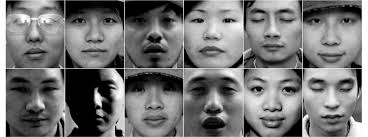Figure 2. Examples of CAS-PEAL dataset face images.

CAS-PEAL was divided into two sets, i.e. training set and test set. The training set was randomly selected from 800 face images of 200 different individuals under non-standard lighting conditions (lighting subset), 400 face images of 100 individuals with non-standard face expressions (expression subset) and 80 occluded face images of 20 different individuals with accessory such as glasses (accessory subset). Then, standard face images of randomly selected non-standard face images were also added to the training set. The test face images were selected from expression subset (expression), lighting subset (lighting), accessory subset (accessory), non-standard background face images subset (background), individual face images taken at different ages (Time), individual face images with non-standard distance from the camera (distance), and individual face images with hats (hat).

Figure 3 shows the sensitivity of overall accuracy of our proposed method to the parameter γ. As it can be seen, the accuracy of our proposed model for γ = 100 is the best. According to Table 1, the overall accuracy of KED for the best value of its hyper-parameter, i.e. for γ=0.01, is 93.47%, while the overall accuracy of our proposed method for the best value of its hyper-parameters, i.e. for γ = 100 and θ=105, is 94.41% which is better than that of KED method. In addition, the speed of our proposed model is better than that of KED.

## 3.png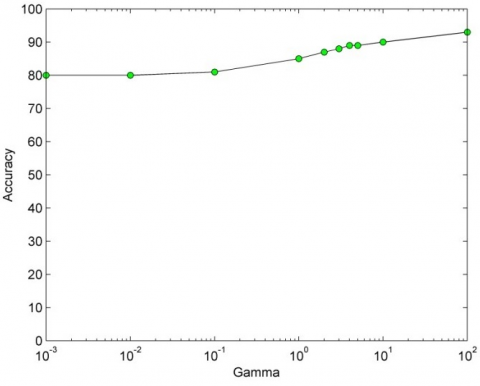Figure 3. Sensitivity of the overall accuracy of our proposed model to the parameter Gamma (γ)

Table 1. Overall accuracy and speed of our proposed model and KED for the whole test set

 method Running time (sec.) Overall accuracy (%) KED 967 93.47 proposed 888 94.41

## 4.png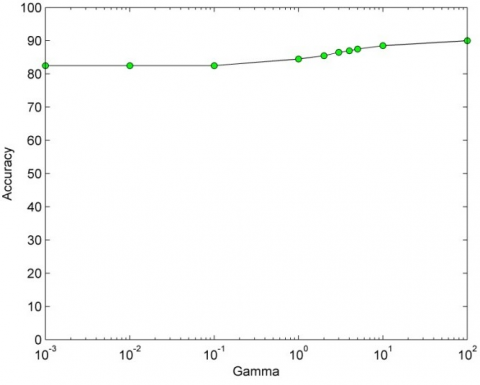Figure 4. Sensitivity of the accuracy of our proposed model to the parameter Gamma (γ) for “Accessory” subset

## 5.png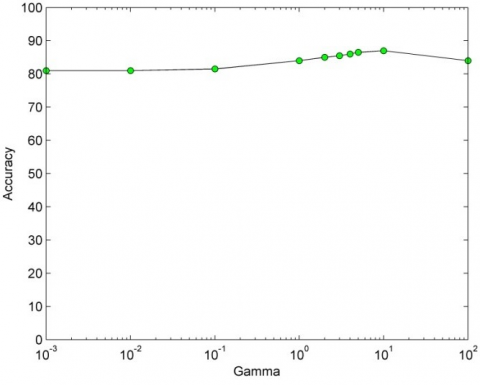Figure 5. Sensitivity of the accuracy of our proposed model to the parameter Gamma (γ) for “Lighting” subset

## 6.png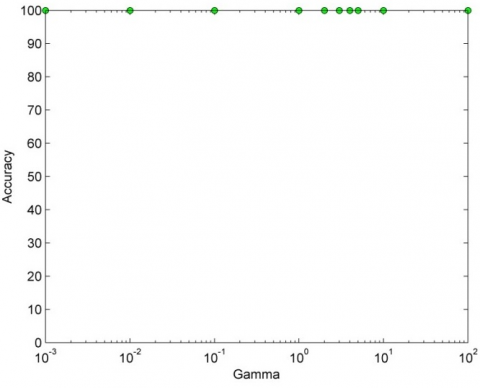Figure 6. Sensitivity of the accuracy of our proposed model to the parameter Gamma (γ) for “Expression” subset

## 7.png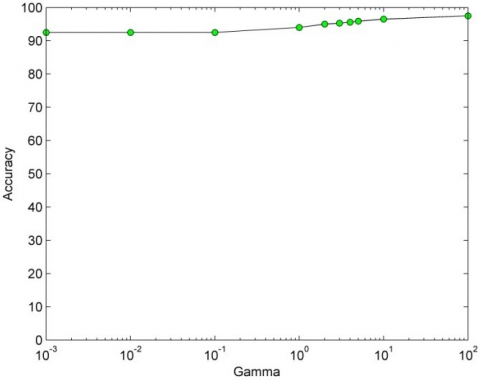Figure 7. Sensitivity of the accuracy of our proposed model to the parameter Gamma (γ) for “Sunglass” subset

## 8.png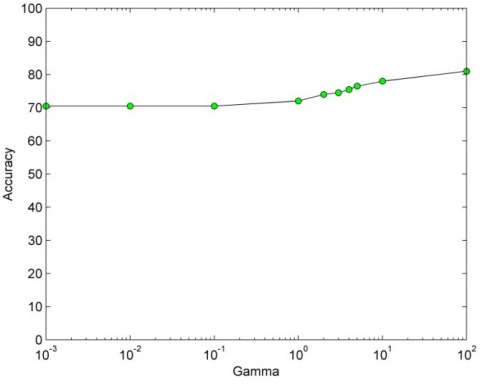Figure 8. Sensitivity of the accuracy of our proposed model to the parameter Gamma (γ) for “Hat” subset

Table 2. Accuracy of our proposed model, SRC, ESRC, KDA+SRC, KDA+ESRC, LED, and KED for different test data subsets

 Method Test data subset Expression Lighting Hat Accessory SRC 98.21 17.31 51.17 72.87 ESRC 99.70 82.08 75.66 87.05 KDA +SRC 99.69 82.73 65.85 80.81 KDA+ESRAC 99.69 83.03 65.93 80.85 LED 99.12 66.83 72.26 84.78 KED 99.80 84.55 86.62 92.94 Proposed 99.80 86.06 89.66 94.44

Figures 4-8 show the sensitivity of accuracy of our proposed method to the parameter γ for different subsets of dataset. As it can be seen, the accuracy of our proposed model is not too sensitive to the parameter γ, specially for some test subsets such as “Expression”. Table 2 compares the accuracy of our proposed model with SRC, ESRC, KDA+SRC, KDA+ESRC, LED, and KED for different test data subsets. Table 3 compares the best obtained accuracy of the proposed method with 1NN, SSEC, RRC, SLF-RKR, MOST, KED methods. As can be seen, the accuracy of the proposed method for any type of test image, except for the distance, is better or equal to the accuracy of the other methods.

Table 3. Accuracy of our proposed model, 1NN, SSEC, RRC, SLF-RKR, MOST, KED for different test data subsets

 Method Test data subset Distance Background Time Expression Lighting Accessory 1NN 76.91 39.69 38.79 80.31 2.874 38.75 SSEC 84.23 66.83 51.94 74.51 17.39 66.64 RRC 97.90 95.64 96.67 93.98 29.32 84.19 SLF-RKR 99.69 99.88 98.48 99.64 28.81 90.88 MOST 99.75 99.01 97.88 98.15 82.39 80.35 KED 100 100 98.48 99.80 84.55 92.94 Proposed 99.89 100 100 99.80 86.06 94.44
5. Conclusion

The kernel extended dictionary learning model (KED) is a new type of SRC, which represents the test datum as a linear combination of dictionary set and extended dictionary set. There were four criticisms to make about KED. To address these four problems, a novel model was proposed in this paper. Experimental results on real dataset showed that the accuracy of the proposed model is better than that of KED, SRC, ESRC, KDA+SRC, KDA+ESRC, LED, 1NN, SSEC, RRC, SLF-RKR, MOST, and runtime of the proposed model is at-least better than that of KED.

Nomenclature
 X Standard face image processed using LBP $\dddot{X}$ Occluded face image processed using LBP y Test face image processed using LBP yKDA Processed test face image in KDA space $\mu_{\mathrm{KDA}}$ Occluded images mean in the KDA space $\dddot{D}$ Principle components of occlusion variation (Extended dictionary) $\ddot{\Lambda}$ Eigen values of occlusion variation $\widetilde{D}$ Basic dictionary $\gamma, \theta$ Hyper-parameters of our proposed model $\mathrm{k}(., .)$ Kernel Gaussian function
References

 Wright, J., Yang, A.Y., Ganesh, A., Sastry, S., Ma, Y. (2009). Robust face recognition via sparse representation. IEEE Transactions on Pattern Analysis and Machine Intelligence, 31(2): 210-227. https://doi.org/10.1109/TPAMI.2008.79

 He, R., Zheng, W.S., Hu, B.G. (2011). Maximum correntropy criterion for robust face recognition. IEEE Transactions on Pattern Analysis and Machine Intelligence, 33(8): 1561-1576. https://doi.org/10.1109/TPAMI.2010.220

 He, R., Zheng, W.S., Tan, T.N., Sun, Z.N. (2014). Half-quadratic-based iterative minimization for robust sparse representation. IEEE Transactions on Pattern Analysis and Machine Intelligence, 36(2): 261-275. https://doi.org/10.1109/TPAMI.2013.102

 Yin, J., Liu, Z.H., Jin, Z., Yang, W.K. (2012). Kernel sparse representation based classification. Neurocomputing. Neurocomputing, 77(1): 120-128. https://doi.org/10.1016/j.neucom.2011.08.018

 Fan, Z., Wei, C. (2020). Fast kernel sparse representation based classification for Undersampling problem in face recognition. Multimedia Tools and Applications, 79(11): 7319-7337. https://doi.org/10.1007/s11042-019-08211-x

 Fan, Z.Z., Zhang, D., Wang, X., Zhu, Q., Wang, Y.F. (2018). Virtual dictionary based kernel sparse representation for face recognition. Pattern Recognition, 76: 1-13. https://doi.org/10.1016/j.patcog.2017.10.001

 Shu, T., Zhang, B., Tang, Y.Y. (2020). Sparse supervised representation-based classifier for uncontrolled and imbalanced classification. IEEE Transactions on Neural Networks and Learning Systems, 31(8): 2847-2856. https://doi.org/10.1109/TNNLS.2018.2884444

 Deng, W., Hu, J., Guo, J. (2012). Extended SRC: undersampled face recognition via intraclass variant dictionary. IEEE Transactions on Pattern Analysis and Machine Intelligence, 34(9): 1864-1870. https://doi.org/10.1109/TPAMI.2012.30

 Dong, X., Zhang, H.X., Zhu, L., Wan, W.B., Wang, Z.H., Wang, Q., Guo, P.L., Ji, H., Sun, J. (2019). Collaborative representation for face recognition based on bilateral filtering. International Journal of Computer Science, 58: 187-194.

 Akhtar, N., Shafait, F., Mian, A. (2017). Efficient classification with sparsity augmented collaborative representation. Pattern Recognition, 65: 136-145. https://doi.org/10.1016/j.patcog.2016.12.017

 Liu, Q., Liu, C. (2017). A novel locally linear KNN method with applications to visual recognition. IEEE Transactions on Neural Networks and Learning Systems, 28(9) 2010-2021. https://doi.org/10.1109/TNNLS.2016.2572204

 Huang, K.K., Dai, D.Q., Ren, C.X., Zhao, R.L. (2017). Learning kernel extended dictionary for face recognition. IEEE Transactions on Neural Networks and Learning Systems, 28(5): 1082-1094. https://doi.org/10.1109/TNNLS.2016.2522431

 Liu, W., Wang, Y., Li, S. (2011). LBP feature extraction for facial expression recognition. Journal of Information & Computational Science, 8(3): 10.

 Mika, S. (1999). Fisher discriminant analysis with Kernels. IEEE Conference on Neural Networks for Signal Processing IX, Madison, WI, USA, pp. 41-48. https://doi.org/10.1109/NNSP.1999.788121

 Wold, S., Esbensen, K., Geladi, P. (1987). Principal component analysis. Chemometrics and Intelligent Laboratory Systems, 2(1-3): 37-52. https://doi.org/10.1016/0169-7439(87)80084-9

 Zhang, L., Yang, M, Feng, X. (2011). Sparse representation or collaborative representation: Which helps face recognition? IEEE. International Conference on Computer Vision, Barcelona, Spain, pp. 471-478. https://doi.org/10.1109/ICCV.2011.6126277

 Yang, A., Ganesh, A., Sastry, S., Ma, Y. (2010). Fast ℓ1-minimization algorithms and an application in robust face recognition. EECS Department, University of California, Berkeley.